# General var get_follow_token = function(successCallback) { \$.ajax({ dataType: "json", url: '/matlabcentral/profile/api/v1/following//token' }).success(function (data) { successCallback(data['su_token']); }); } // Group follow to always return Discussion or group followed_type even for Polls \$("#follow_group_link").click(function() { var span = \$("#follow_group_link").find('span').first(); \$("#follow_group_link").html('<span class="icon-spinner add_rotating"></span>'); get_follow_token(function(token) { if (span.attr('data-action') == 'follow') { \$.post('/matlabcentral/profile/api/v1/following//create', {followed_type: 'Discussion', followed_id: 'general', title: "General", contributor: false, su_token: token, content_url: "https://www.mathworks.com/matlabcentral/discussions/general"}) .done(function() { setGroupFollowed(); }) } else { \$.post('/matlabcentral/profile/api/v1/following//destroy', {followed_type: 'Discussion', followed_id: 'general', su_token: token, content_url: "https://www.mathworks.com/matlabcentral/discussions/general"}) .done(function() { setGroupUnfollowed(); }) }; }); }); function setGroupFollowed() { \$("#follow_group_link").html('<span class="follow_icon icon-remove-circle" data-action="unfollow"></span> Arrêter de suivre'); \$('#group_follow_confirmation').show(250); \$('#followedNavDesktop').removeClass('hidden'); \$('#fol_chan_general').removeClass('hidden'); \$('#chan_general').addClass('hidden'); }; function setGroupUnfollowed() { \$("#follow_group_link").html('<span class="follow_icon icon-add-circle" data-action="follow"></span> Suivre'); \$('#group_follow_confirmation').hide(250); \$('#fol_chan_general').addClass('hidden'); \$('#chan_general').removeClass('hidden'); };

Kittiwith
Last activity environ 3 heures il y a

### Error using plannerBiRRT/plan

This is my code
%send food and medicine in flood situation
% 1. create map
clc
clear
tic
map3D = occupancyMap3D; % create space for
[xGround,yGround,zGround] = meshgrid(0:240,0:350, 0); %set floor x=500 y=500
xyzGround = [xGround(:) yGround(:) zGround(:)]; %use for mergegrid
occval = 0;
setOccupancy(map3D,xyzGround,occval)
% xspace = 20 y = 45 create
[xB1, yB1, zB1] = meshgrid(50:130, 60:110, 0:20); %unionmall
[xB2, yB2, zB2] = meshgrid(80:250, 160:240, 0:30); %centralladprao
[xB3, yB3, zB3] = meshgrid(220:250, 290:340, 0:60); %centarahotel
[xB4, yB4, zB4] = meshgrid(0:30, 10:40, 0:80); %condo
[xB5, yB5, zB5] = meshgrid(150:170, 80:100, 0:10); %house1
[xB6, yB6, zB6] = meshgrid(180:200, 80:100, 0:10); %house2
[xB7, yB7, zB7] = meshgrid(210:230, 80:100, 0:10); %house3
[xB11, yB11, zB11] = meshgrid(240:270, 80:100, 0:10); %house7
[xB8, yB8, zB8] = meshgrid(150:170, 50:70, 0:10); %house4
[xB9, yB9, zB9] = meshgrid(180:200, 50:70, 0:10); %house5
[xB10, yB10, zB10] = meshgrid(210:230, 50:70, 0:10); %house6
[xB12, yB12, zB12] = meshgrid(240:270, 50:70, 0:10); %house8
[xB13, yB13, zB13] = meshgrid(200:260, 0:30, 0:13); %villageoffice
xyzB = [xB1(:), yB1(:), zB1(:);
xB2(:), yB2(:), zB2(:);
xB3(:), yB3(:), zB3(:);
xB4(:), yB4(:), zB4(:);
xB5(:), yB5(:), zB5(:);
xB6(:), yB6(:), zB6(:);
xB7(:), yB7(:), zB7(:);
xB8(:), yB8(:), zB8(:);
xB9(:), yB9(:), zB9(:);
xB10(:), yB10(:), zB10(:);
xB11(:), yB11(:), zB11(:);
xB12(:), yB12(:), zB12(:);
xB13(:), yB13(:), zB13(:);];
obs = 0.65; % ;=not show in command window
my3Dmap = map3D;
updateOccupancy(my3Dmap,xyzB,obs);
%show(my3Dmap);
% 2. distination
%my3Dmap.FreeThreshold = my3Dmap.OccupiedThreshold;
startPose = [50 60 25 pi/2;
250 310 65 pi/2;
100 170 35 pi/2;
100 30 85 pi/2;
155 90 15 pi/2;
250 60 15 pi/2;
160 60 15 pi/2
220 15 20 pi/2;];
goalPose = startPose;
ss = ExampleHelperUAVStateSpace("MaxRollAngle",pi/3,...
"AirSpeed",5,...
"FlightPathAngleLimit",[-0.5 0.5],...
"Bounds",[-10 400;-10 4000; -10 100; -pi pi]);
%threshold = [(goalPose-0.5)' (goalPose+0.5)'; -pi pi];
%setWorkspaceGoalRegion(ss,goalPose,threshold)
sv = validatorOccupancyMap3D(ss,"Map",my3Dmap);
sv.ValidationDistance = 0.5;
planner = plannerBiRRT(ss,sv);
planner.MaxConnectionDistance = 100;
%planner.GoalBias = 0.10;
%1,0.1,0.15,0.5,0.2,0.13,
planner.MaxIterations = 100000;
%planner.GoalReachedFcn = @(~,x,y)(norm(x(1:3)-y(1:3)) < 5);
planner.MaxNumTreeNodes = 10000;
numPose = length(startPose);
distances = zeros(numPose, numPose);
for i = 1:numPose
for j = 1:numPose
if i ~= j
rng(100, "twister");
start = [startPose(i,1), startPose(i,2), startPose(i,3), startPose(i,4)];
goal = [goalPose(j,1), goalPose(j,2), goalPose(j,3), goalPose(j,4)];
[pthObj, solnInfo] = plan(planner, start, goal);
smoothWaypointsObj = exampleHelperUAVPathSmoothing(ss, sv, pthObj);
distances(i, j) = pathLength(smoothWaypointsObj);
end
end
end
toc
and the error belowGumaa Abdelrhim
Last activity 40 minutes il y a

### Moment- axial force intrection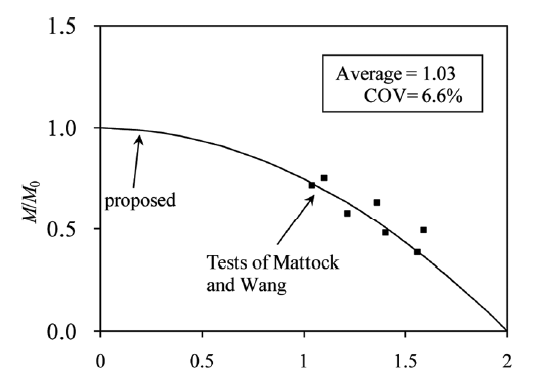Hi, I have this equation for moment-axial force interaction: 0.25*(M/M0)^2 + (N/N0) = 1. This equation produces the following curve. Now, I need to make a change to this equation so that the first term becomes (M/M0)^2, and the second term can be changed while keeping (N/N0). The other side of the equation remains the same. ((M/M0)^2+?? = 1). I need to get the same curve as in the figure.
Yoann
Last activity environ 14 heures il y a

### ultrasound video clip analysis

...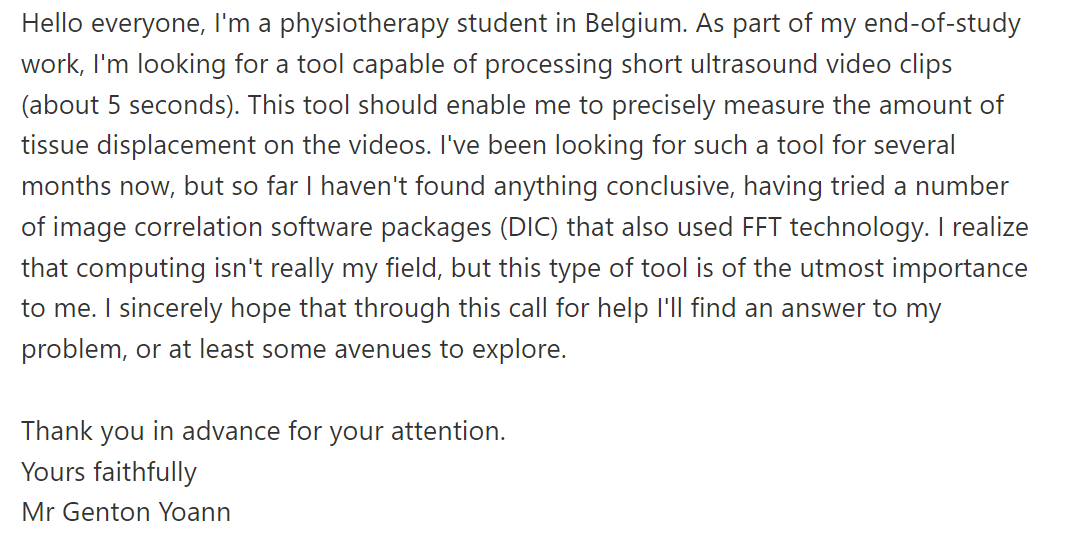Ghg
Last activity le 10 Déc 2023 à 8:17

### I need solutions for this three tasks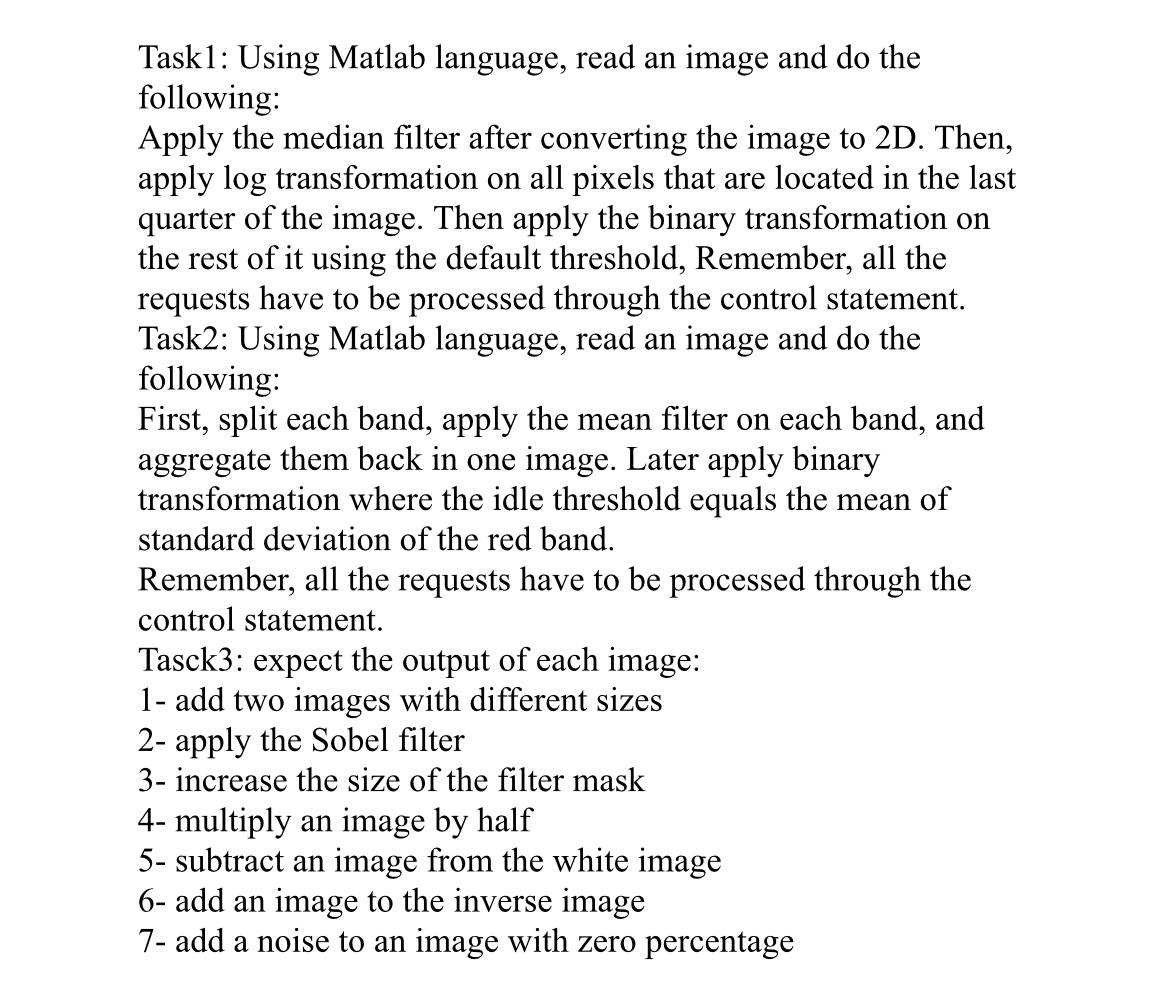Kheng Seng
Last activity le 9 Déc 2023 à 9:37

### How to model combustion chamber or heater for gas turbine system using Simulink?

Hello everyone,
I am trying simulate a gas turbine system for my course on modelling and optimization of energy system. I have come out with a design consisting of a compressor, air preheater, combustion chamber and gas turbine. When I try to do the modelling in Simulink, I couldnt find something similar to combustion chamber. Does anyone have any experience in doing this?
Orginal Design:I have also amended it and come out with second design, where I change to combustion chamber to a heater and the exhaust air is recycled back to air compressor, making it a closed loop system. But I am also unable to find heater from the simulink library, the closest i can get is convective heat transfer. Can someone help on this too and if the closed loop system is feasible to model?
Design 2 :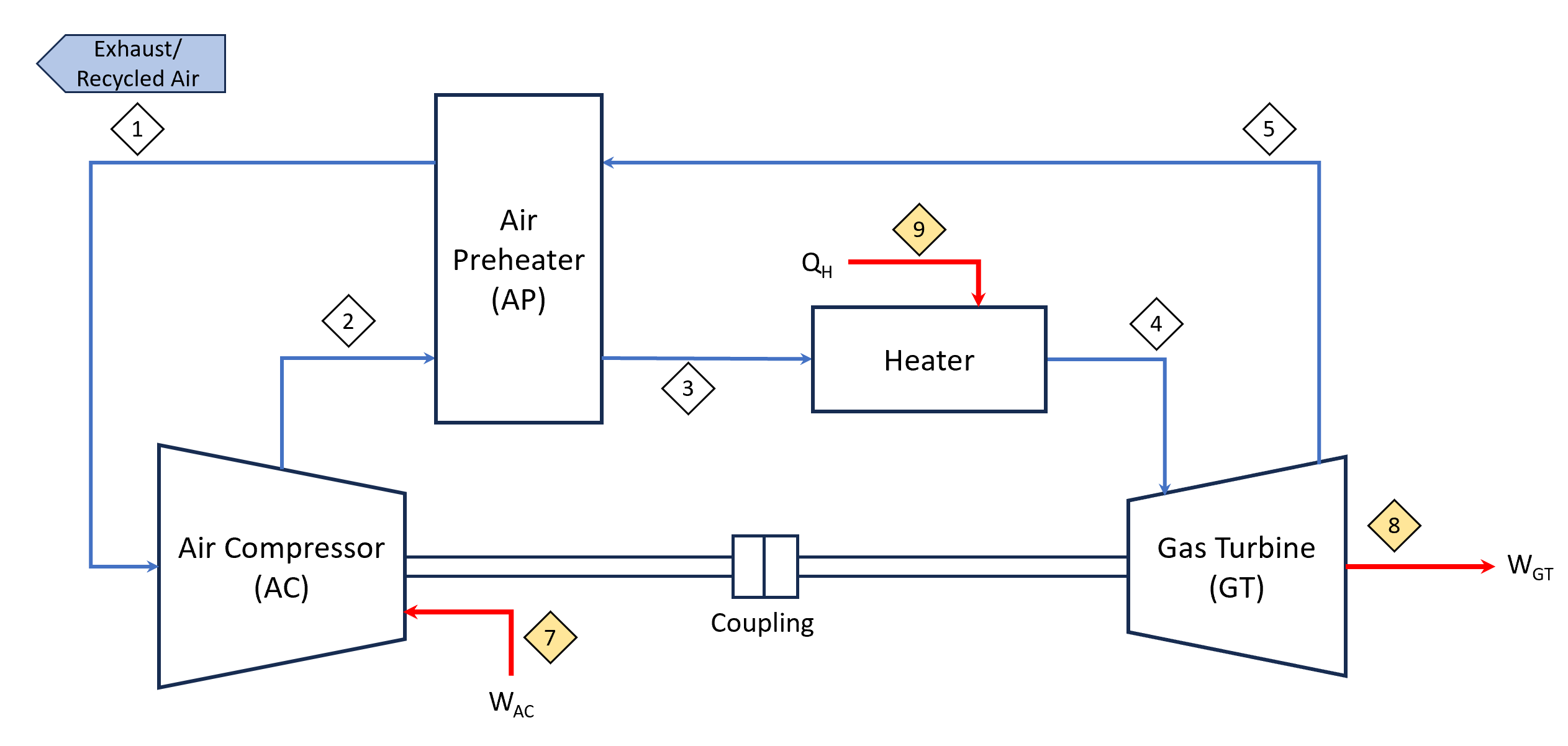Riccardo Spartà
Last activity environ 5 heures il y a

### How to get real-time outputs and real-time inputs from/to Simulink, without using Simulink Real-Time?

Hi everyone,
for my thesis project I would need to get, every second of the simulation, real-time output data from a Simulink model, without using Simulink Real-Time, as it supports only Speedgoat Hardware. Is there a particular set of blocks for that purpose?
I have to provide that real-time data to hardware for an Hardware in the Loop Simulation so I will need to be able also to receive, as an input, data from the hardware to the Simulink model, again every second of the simulation, to close the loop of the simulation.
Sushil Kunwar
Last activity le 8 Déc 2023 à 1:44

### Curve line plot

Hi! I am using matlab inbuilt plot option( without typing code) to plot the data. This joins the data points using straight line by default. What will be the easiest way to plot if I want curve to join the points instead?
Andi
Last activity le 7 Déc 2023 à 1:44

### Library 'Servo' is not uploaded to the board ERROR

I keep getting this error message when trying to run my code, I'm confident my wiring is correct and I do not know what this error means-
Library 'Servo' is not uploaded to the board. Clear the current Arduino object and recreate it to include the appropriate library. For a list of available libraries, type 'listArduinoLibraries'.
here is my code
a=arduino();
s1 = servo(a, 'D9', 'MinPulseDuration', 700*10^-6, 'MaxPulseDuration', 2300*10^-6);
writePosition(s1,0);
userResponse = "yes";
while userResponse == "yes"
if voltage < 1.67155
resistor = "47k";
writePosition(s1,0.25);
minR = 47000 * 0.95;
maxR = 47000 * 1.05;
elseif voltage < 3.53128
resistor = "10k";
writePosition(s1,0.5);
minR = 10000 * 0.95;
maxR = 10000 * 1.05;
elseif voltage < 4.69941
resistor = "1k";
writePosition(s1,0.75);
minR = 1000 * 0.95;
maxR = 1000 * 1.05;
else
resistor = "330";
writePosition(s1,1);
minR = 330 * 0.95;
maxR = 330 * 1.05;
end
resistance = voltageToResistance(voltage);
withinTolerance = (resistance >= minR && resistance <= maxR);
disp(resistor)
if withinTolerance == 1
writeDigitalPin(a, "D4", 1);
writeDigitalPin(a, "D5", 0);
%disp("green")
else
writeDigitalPin(a, "D4", 0);
writeDigitalPin(a, "D5", 1);
%disp("Red")
end
userResponse = input("Would you like to check another resistor? ","s");
writePosition(s1,0);
writeDigitalPin(a, "D4", 0);
writeDigitalPin(a, "D5", 0);
end
Siebe Caems
Last activity le 4 Déc 2023 à 14:58

### Difference rotating over moving angle and normal angle

Hello,
What is the difference between a moving angle and a normal angle?
Compute the Rotation about Y-axis over moving angle a = 30°
then
rotation about X-axis of angle β= 45°
What will the code like?
Thank you
Engineer
Last activity le 30 Nov 2023 à 12:13

### Error C MEX S-function

Hello there, I have a Amesim Black box model in Simulink. On my desktop, it works well. Also i tried to run on other desktops. Most of them work well. But some of them gives following error: Error in 'Model' while executing C MEX S-function 'Model', (mdlInitializeConditions), at time 0.0. Caused by: in Simcenter Amesim mexfunction: 'Model', Simcenter Amesim fatal error Cannot initialize Simcenter Amesim Model. EXIT This is an urgent case for me. Could you please help me?

Thank you so much.

Varalakshmi Suresh
Last activity le 30 Nov 2023 à 7:19

Dear Community,
I am trying to perform PowerGUI Loadflow analysis for EV Charging station. The model converges but the load flow vaues on the DC bus is zero. Whether power_loadflow supports Mixed AC/DC load Flow.
Can someone help me by providing guidance on how to preform the same
Regards
Vara
Ayush
Last activity le 29 Nov 2023 à 15:10

### facing an issue in

we have extracted a .xml file from solidworks to import in matlab, when we tried to import it shows that simscape multibody should be installed and when we tried to install it it shows the message attached below.
we are working on an educational license provided by our university, is it the issue?
Bewar Yousif Ali
Last activity environ 10 heures il y a

### How to fix this problem 0^0 in Matlab !?

It is crucial to understand that this expression could be used in problems related to engineering, physics, mathematics, or any other aspect of real life.
Typically, Matlab is used to solve PDE and ODE problems. Perhaps users calculated this term 0^0 incorrectly in the process.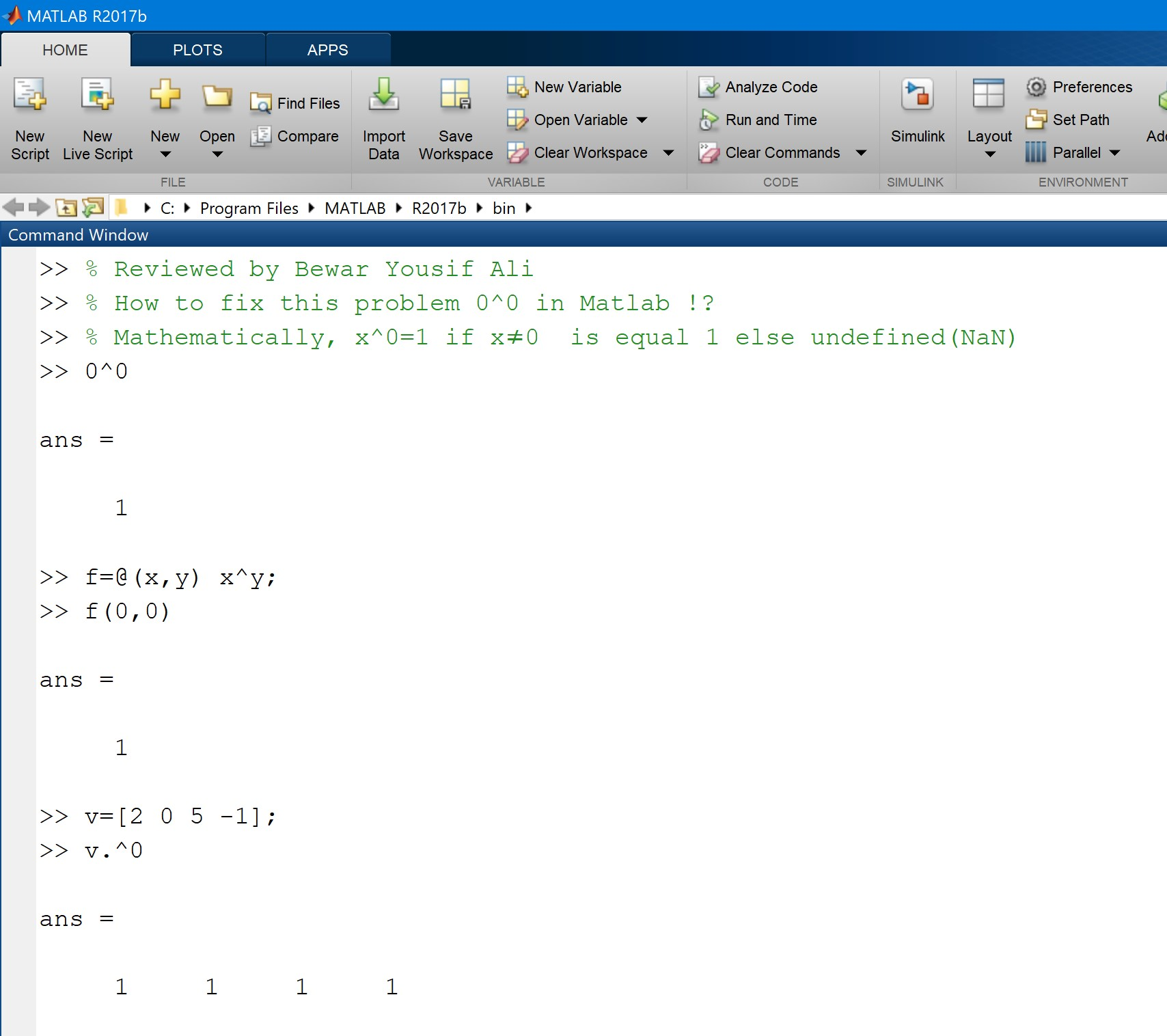>> % Reviewed by Bewar Yousif Ali
>> % How to fix this problem 0^0 in Matlab !?
>> % Mathematically, x^0=1 if x≠0 is equal 1 else undefined(NaN)
>> 0^0
ans =
1
>> f=@(x,y) x^y;
>> f(0,0)
ans =
1
>> v=[2 0 5 -1];
>> v.^0
ans =
1 1 1 1
JUAN CARLOS
Last activity environ 10 heures il y a

### i=dsolve('Dy=-8*y+40*sin(8*t)','y(0)=5') Warning: Support for character vector or string inputs will be removed in a future release. Instead, use syms to declare variables and replace inputs such as dsolve('Dy = -3*y') with syms y(t); dsolve(diff(y,

i=dsolve('Dy=-8*y+40*sin(8*t)','y(0)=5')
Warning: Support for character vector or string inputs will be
removed in a future release. Instead, use syms to declare
variables and replace inputs such as dsolve('Dy = -3*y') with syms
y(t); dsolve(diff(y,t) == -3*y). > In dsolve (line 126)
Sanika Patil
Last activity le 25 Nov 2023 à 4:39

### face recognition GUI

Error using matlab.ui.control.internal.controller.ComponentController/executeUserCallback Error while evaluating Button PrivateButtonPushedFcn.
help to solve this error in face recognition GUI
Joseph
Last activity le 8 Déc 2023 à 16:26

### Phone motion and orientation calculation?

Last activity le 23 Nov 2023 à 9:25

### 打开matlab 示例 提示错误Shaunak Deshpande
Last activity le 20 Nov 2023 à 20:21

### Error using tall/cellfun

I'm getting an error error "using tall/cellfun" while doing a project listed in mathworks "Denoise Speech Using Deep Learning Networks". I don't know how to fix this error pr why this error occured in the first place. Seeking explanation for this particuar error. If you know anuthing about it then please consider helping me below this post.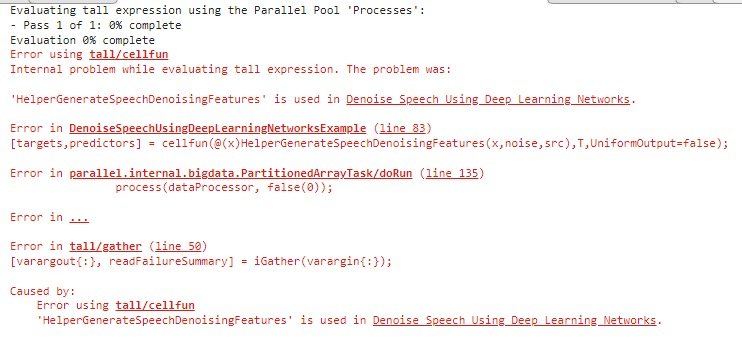Last activity le 5 Déc 2023 à 8:27

### Two-phase flow solenoid valve

Hello, I want to use a solenoid valve to open and close the two-phase flow circuit, and I should use those elements to achieve it.